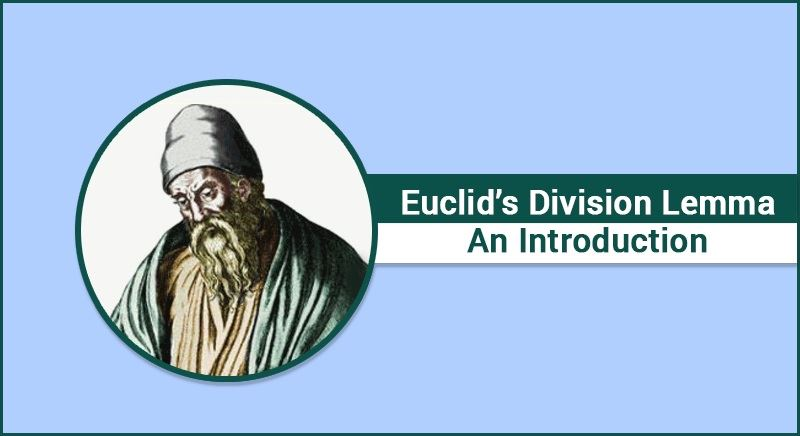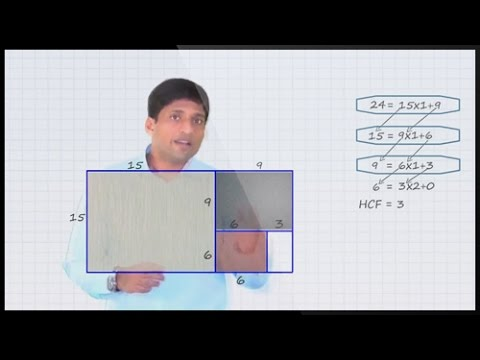# Euclid's Division Lemma: An Introduction

According to Euclid’s Division Lemma if we have two positive integers a and b, then there exist unique integers q and r which satisfies the condition a = bq + r where 0 ≤ r ≤ b.

The basis of the Euclidean division algorithm is Euclid’s division lemma. To calculate the Highest Common Factor (HCF) of two positive integers a and b we use Euclid’s division algorithm. HCF is the largest number which exactly divides two or more positive integers. By exactly we mean that on dividing both the integers a and b the remainder is zero.Let us now get into the working of this euclidian algorithm.

Consider we have two numbers 78 and 980 and we need to find the HCF of both of these numbers. To do this, we choose the largest integer first, i.e. 980 and then according to Euclid Division Lemma, a = bq + r where 0 ≤ rb;

980 = 78 × 12 + 44

Now, here a = 980, b = 78, q = 12 and r = 44.

Now consider the divisor as 78 and the remainder 44 and apply the Euclid division method again, we get

78 = 44 × 1 + 34

Similarly, consider the divisor as 44 and the remainder 34 and apply the Euclid division method again, we get

44 = 34 × 1 + 10

Following the same procedure again,

34 = 10 × 3 + 4

10=4×2+2

4=2×2+0

As we see that the remainder has become zero, therefore, proceeding further is not possible and hence the HCF is the divisor b left in the last step which in this case is 2. We can say that the HCF of 980 and 78 is 2.

Let us try another example to find the HCF of two numbers 250 and 75. As the larger integer is 250, therefore, applying Euclid Division Lemma a = bq + r where 0 ≤ r ≤ b, we have

a = 250 and b = 75

⇒ 250 = 75 × 3 + 25

Applying the Euclid’s Division Algorithm again we have,

75 = 25 × 3 + 0

As the remainder becomes zero, we cannot proceed further. According to the algorithm, divisor in this case which is 25 here is the HCF of 250 and 75.

Example: Find the HCF of 81 and 675 using the Euclidean division algorithm.

Solution: The larger integer is 675, therefore, applying the Division Lemma a = bq + r where 0 ≤ r ≤ b, we have

a = 675 and b = 81

⇒ 675 = 81 × 8 + 27

Applying the Euclid’s Division Algorithm again we have,

81 = 27 × 3 + 0

We cannot proceed further as the remainder becomes zero. According to the algorithm divisor, in this case, is 27 which is the HCF of 675 and 81.

This algorithm has got many practical applications in finding the properties of numbers. There is a lot more left to learn in real numbers. Enrich your knowledge by visiting our website www.byjus.com and download BYJU’s-the learning app and learn anywhere.

Recent Articles